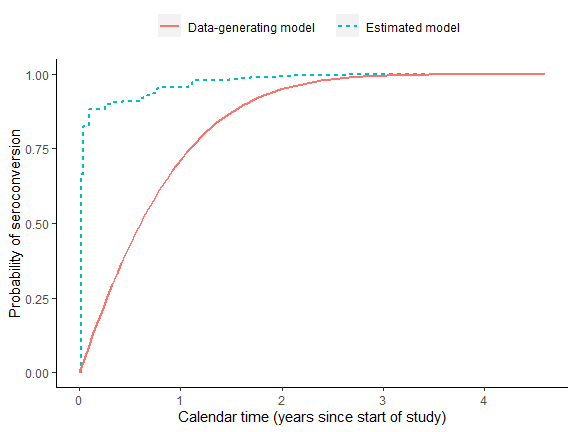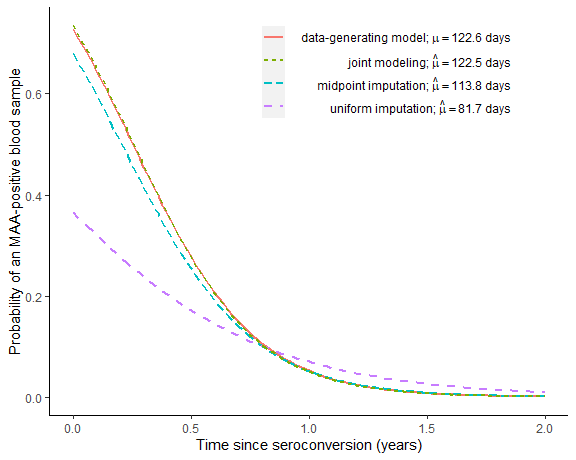# how-to-use-rwicc

This vignette shows how to generate a simulated data set and analyze it using the model and estimation algorithm described in “Regression with Interval-Censored Covariates: Application to Cross-Sectional Incidence Estimation” by Morrison, Laeyendecker, and Brookmeyer (2021) in Biometrics: https://onlinelibrary.wiley.com/doi/10.1111/biom.13472.

First, we simulate some data:


set.seed(1)

library(rwicc)
theta_true = c(0.986, -3.88)
hazard_alpha = 1
hazard_beta = 0.5
sim_data = simulate_interval_censoring(
"theta" = theta_true,
"study_cohort_size" = 4500,
"preconversion_interval_length" = 365,
"hazard_alpha" = hazard_alpha,
"hazard_beta" = hazard_beta)

# extract the participant-level and observation-level simulated data:
sim_participant_data = sim_data$pt_data sim_obs_data = sim_data$obs_data
rm(sim_data)

Here’s a look at the first few rows of participant-level data:

library(pander)
pander(head(sim_participant_data))
ID E L R
1 2001-06-16 2001-06-16 2002-06-17
2 2001-05-09 2001-05-09 2002-05-06
3 2001-10-26 2001-10-26 2002-10-30
4 2001-09-27 2001-09-27 2002-09-25
5 2001-07-06 2001-07-06 2002-06-29
6 2001-11-03 2001-11-03 2002-11-02
• E is the individual’s enrollment date
• L is the date of the last HIV-negative test
• R is the date of the first HIV-positive test

Next, let’s look at the first few rows of observation-level (longitudinal) data:

pander(head(sim_obs_data))
ID O Y
1 2002-06-17 1
1 2002-07-15 0
1 2002-08-12 1
1 2002-09-09 0
1 2002-12-02 0
1 2003-02-24 0
• O is the observation date
• Y is the MAA classification (1 = “recent infection”, 0 = “long-term infection”)

The two tables are linked by the variable ID.

Now, we will apply our proposed analysis (this takes a couple of minutes; use argument verbose = TRUE to print progress messages):

EM_algorithm_outputs = fit_joint_model(
obs_level_data = sim_obs_data,
participant_level_data = sim_participant_data,
bin_width = 7,
verbose = FALSE)

The output of fit_joint_model() is a list with several components:

names(EM_algorithm_outputs)
#>  "Theta"               "Mu"                  "Omega"
#>  "converged"           "iterations"          "convergence_metrics"

Theta is the vector of estimated logistic regression coefficients for $$P(Y|T)$$ (intercept and slope):


pander(EM_algorithm_outputs$Theta) (Intercept) T 1.019 -3.953 Mu is the corresponding $$\hat{\mu}$$ estimate: mu_est_EM = EM_algorithm_outputs$Mu
print(mu_est_EM)
#>  122.5193

converged indicates whether the algorithm reached its convergence criterion (= 1 if converged, = 0 if not).

EM_algorithm_outputs$converged #>  1 iterations is the number of EM iterations that the algorithm performed: EM_algorithm_outputs$iterations
#>  112

convergence_metrics gives the values of all four metrics that we might use to evaluate convergence:

• diff logL: change in log-likelihood between iterations
• diff mu: change in $$\hat{\mu}$$
• max abs diff coefs: $$\max_{j\in 0:1} \{|\hat{\theta}_j^{(k)} - \hat{\theta}_j^{(k-1)}|\}$$
• max abs rel diff coefs: $$\max_{j\in 0:1} \{|(\hat{\theta}_j^{(k)} - \hat{\theta}_j^{(k-1)})/\hat{\theta}_j^{(k-1)}|\}$$

By default, the convergence criterion is: diff logL < 0.1 and max abs rel diff coefs < 0.0001.

pander(EM_algorithm_outputs$convergence_metrics) diff logL diff mu max abs diff coefs max abs rel diff coefs 0.008769 0.008171 0.0001012 9.931e-05 Next, we perform an alternative analysis using midpoint imputation:  theta_est_midpoint = fit_midpoint_model( obs_level_data = sim_obs_data, participant_level_data = sim_participant_data ) pander(theta_est_midpoint) (Intercept) T_midpoint 0.7572 -3.662 Here, we perform an alternative analysis using uniform imputation: # uniform imputation: theta_est_uniform = fit_uniform_model( obs_level_data = sim_obs_data, participant_level_data = sim_participant_data ) pander(theta_est_uniform) theta0 theta1 -0.549 -2.037 Now, let’s graph the results. First, let’s plot the true and estimated CDFs for the distribution of seroconversion date, for individuals who enroll on the first calendar day of the cohort study:  plot1 = plot_CDF( true_hazard_alpha = hazard_alpha, true_hazard_beta = hazard_beta, omega.hat = EM_algorithm_outputs$Omega)

print(plot1)We can see that our joint modeling approach hasn’t estimated this distribution very accurately for this particular simulated dataset. Nevertheless, the next graph will show us that the joint model very accurately estimates the true distribution $$P(Y|T)$$ and the true value of $$\mu$$:


plot2 = plot_phi_curves(
theta_true = theta_true,
theta.hat_uniform = theta_est_uniform,
theta.hat_midpoint = theta_est_midpoint,
theta.hat_joint = EM_algorithm_outputs\$Theta)

print(plot2)